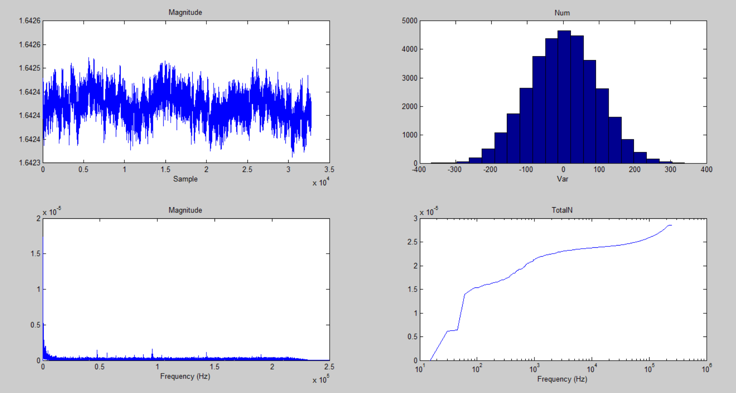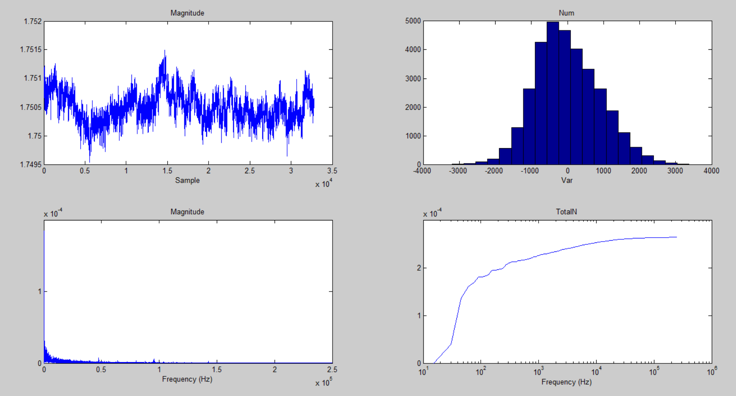# 利用高精密模拟数字转换器评估放大器的噪声性能Avg = mean(num); % 求解样本NUM中的平均值

Std = std(num); % 求解样本NUM的STDEV，就是RMS值

hist(num,20); % 将样本NUM的分布按照直方图绘出

y = fft(num,32768,1); % 对样本NUM进行FFT

y1 = abs(y) / 16384; % 求解频谱分量的幅值

power = y1.^2 * 0.5; % 求解频谱分量的功率

TotalN = sqrt(***(power)); % 将噪声频谱累加